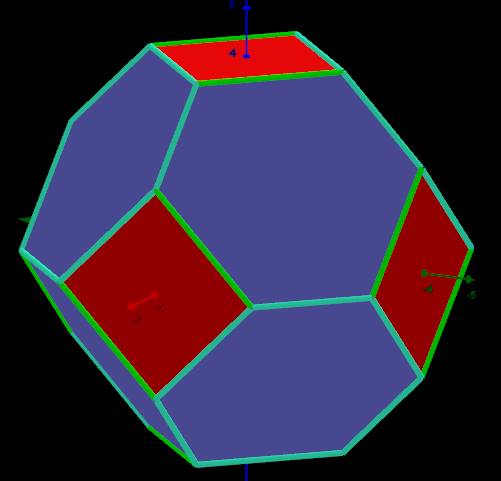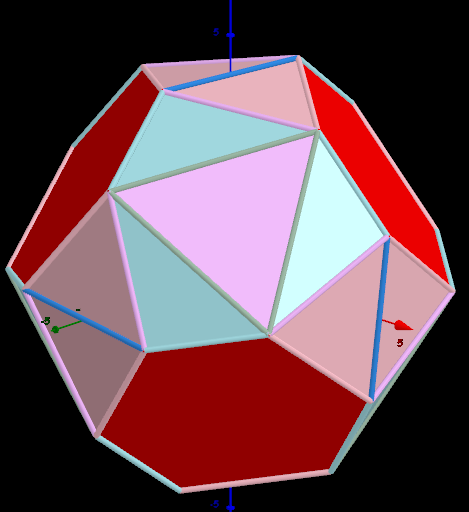# Three-parameter model transformations of the Tetrahedron. Extreme distributions.

Three parameters are used to transform Tetrahedron faces: t - for truncating, q-similarity transformations, α-angle rotation of faces. With these parameters, using the well-known Expansion and Snub operations applied to a polyhedron, you can explore various polyhedra and get a number of Archimedean Solids. The p (distance sum) is defined here as the ratio of the sum of all pairwise distances between the vertices of the polyhedron to the number of these pairs and the radius of circumscribed sphere. Value p can be given the meaning of the mean distance between the vertices of a polyhedron on a unit sphere. There are three parameters that are used for two maximizations of the average distance between vertexes. Difference: in the first case, the angle rotation of faces is fixed(α=0). The buttons should be pressed (iterations) until precision-the difference between the two subsequent values is 10-15. Case 5 corresponds to the first maximization, Case 6- to the second. Iterations are very slow on-line. It is preferable to carry out them off-line for the desktop version. Case 5 is an extreme distribution: ΔφGM=0x100 rad, but Case 6, as can be seen from the table, is not: ΔφGM=2.78x10-3 rad.

## Example 5. Pmax=1.383 075 228 171 583, t, q, α=0 rad, V=24.Case of optimal distribution of points on the surface of a sphere. This means that each of its vertices on a sphere is located at the points of the geometric medians of its other vertices (ΔφGM=5.27*10-9 rad).

## Example 6. t, q, α≠0- Pmax= 1.383 117 342 322 636, V=24Case of nearly optimal distribution of points on the surface of a sphere. This means that each of its vertices on a sphere is located at the points of the geometric medians of its other vertices (ΔφGM=2.78*10-3 rad).